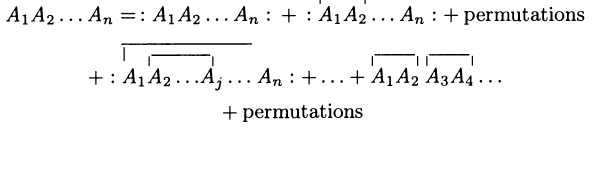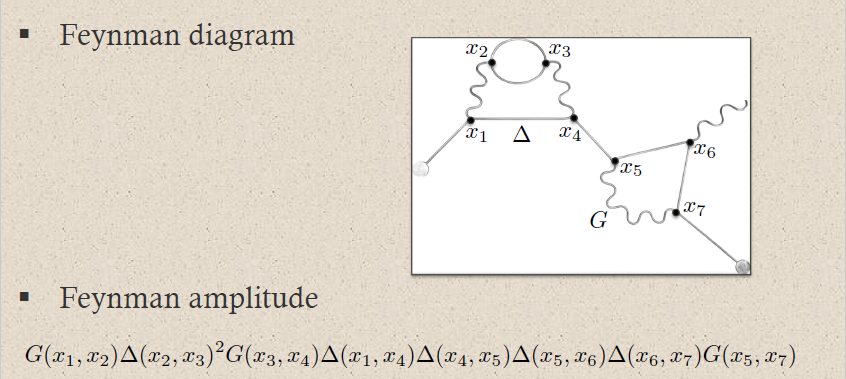Contents

# Contents

## Idea

In relativistic perturbative quantum field theory, the time-ordered product is a product on suitably well-behave observables which re-orders its arguments according to the causal ordering of their spacetime supports befor multiplying with the Wick algebra product.

(Analogously reverse causal ordering this is called the reverse-time ordered or anti-time ordered prouct.)

For example for point-evaluation field observables and distinct events $x,y \in \Sigma$ the time-ordered product is defined by

$T(\mathbf{\Phi}^a(x) \mathbf{\Phi}^b(y)) \;\coloneqq\; \left\{ \array{ \mathbf{\Phi}^a(x) \mathbf{\Phi}^a(y) &\vert& x \, \text{not in the past of}\, y \\ \mathbf{\Phi}^a(y) \mathbf{\Phi}^a(x) &\vert& \text{otherwise} } \right.$

This may be understood as arising from the causal additivity-axiom of the perturbative S-matrix. It generalizes the 1-dimensional time-ordering (path ordering) of the Dyson series in quantum mechanics.

More precisely, the time-ordere product is a commutative algebra-structure on the microcausal polynomial observables of a free Lagrangian field theory equipped with a vacuum state (Hadamard state) which on regular polynomial observables given on the regular polynomial observables by the star product which is induced (via this def.) by the Feynman propagator and which is extended from there, in the sense of extensions of distributions, to all microcausal polynomial observables. (This extension is the “renormalization” of the time-ordered product).

## Definition

### On regular polynomial observables

###### Definition

(time-ordered product on regular polynomial observables)

Let $(E,\mathbf{L})$ be a free Lagrangian field theory over a Lorentzian spacetime and with Green-hyperbolic Euler-Lagrange differential equations; write $\Delta_S = \Delta_+ - \Delta_-$ for the induced causal propagator. Let moreover $\Delta_H = \tfrac{i}{2}\Delta_S + H$ be a compatible Wightman propagator and write $\Delta_F = \tfrac{i}{2}(\Delta_+ + \Delta_-) + H$ for the induced Feynman propagator.

Then the time-ordered product on the space of off-shell regular polynomial observable $PolyObs(E)_{reg}$ is the star product induced by the Feynman propagator (via this prop.):

$\array{ PolyObs(E)_{reg}[ [\hbar] ] \otimes PolyObs(E)_{reg}[ [\hbar] ] &\overset{}{\longrightarrow}& PolyObs(E)_{reg}[ [\hbar] ] \\ (A_1, A_2) &\mapsto& \phantom{\coloneqq} A_1 \star_F A_2 }$

hence

$A_1 \star_F A_2 \; \coloneqq \; ((-)\cdot(-)) \circ \exp\left( \underset{\Sigma \times \Sigma}{\int} \Delta_F^{a b}(x,y) \frac{\delta}{\delta \mathbf{\Phi}^a(x)} \otimes \frac{\delta}{\delta \mathbf{\Phi}^b(y)} \, dvol_\Sigma(x) \, dvol_\Sigma(y) \right)$

(Notice that this does not descend to the on-shell observables, since the Feynman propagator is not a solution to the homogeneous equations of motion.)

###### Proposition

(time-ordered product is indeed causally ordered Wick algebra product)

Let $(E,\mathbf{L})$ be a free Lagrangian field theory over a Lorentzian spacetime and with Green-hyperbolic Euler-Lagrange differential equations; write $\Delta_S = \Delta_+ - \Delta_-$ for the induced causal propagator. Let moreover $\Delta_H = \tfrac{i}{2}\Delta_S + H$ be a compatible Wightman propagator and write $\Delta_F = \tfrac{i}{2}(\Delta_+ + \Delta_-) + H$ for the induced Feynman propagator.

Then the time-ordered product on regular polynomial observables (def. ) is indeed a time-ordering of the Wick algebra product $\star_H$ in that for all pairs of regular polynomial observables

$A_1, A_2 \in PolyObs(E)_{reg}[ [\hbar] ]$

with disjoint spacetime support we have

$T(A_1 A_2) \;=\; \left\{ \array{ A_1 \star_H A_2 &\vert& supp(A_1) {\vee\!\!\!\wedge} supp(A_2) \\ A_2 \star_H A_1 &\vert& supp(A_2) {\vee\!\!\!\wedge} supp(A_2) } \right. \,.$

Here $S_1 {\vee\!\!\!\wedge} S_2$ is the causal order relation (“$S_1$ does not intersect the past cone of $S_2$”). Beware that for general pairs $(S_1, S-2)$ of subsets neither $S_1 {\vee\!\!\!\wedge} S_2$ nor $S_2 {\vee\!\!\!\wedge} S_1$.

###### Proof

Recall the following facts:

1. the advanced and retarded propagators $\Delta_{\pm}$ by definition are supported in the future cone/past cone, respectively

$supp(\Delta_{\pm}) \subset \overline{V}^{\pm}$
2. they turn into each other under exchange of their arguments (this cor.):

$\Delta_\pm(y,x) = \Delta_{\mp}(x,y) \,.$
3. the real part $H$ of the Feynman propagator, which by definition is the real part of the Wightman propagator is symmetric (by definition or else by this prop.):

$H(x,y) = H(y,x)$

Using this we compute as follows:

\begin{aligned} A_1 \underset{\Delta_{F}}{\star} A_2 \; & = A_1 \underset{\tfrac{i}{2}(\Delta_+ + \Delta_-) + H}{\star} A_2 \\ & = \left\{ \array{ A_1 \underset{\tfrac{i}{2}\Delta_+ + H}{\star} A_2 &\vert& supp(A_1) {\vee\!\!\!\wedge} supp(A_2) \\ A_1 \underset{\tfrac{i}{2}\Delta_- + H}{\star} A_2 &\vert& supp(A_2) {\vee\!\!\!\wedge} supp(A_2) } \right. \\ & = \left\{ \array{ A_1 \underset{\tfrac{i}{2}\Delta_+ + H}{\star} A_2 &\vert& supp(A_1) {\vee\!\!\!\wedge} supp(A_2) \\ A_2 \underset{\tfrac{i}{2}\Delta_+ + H}{\star} A_1 &\vert& supp(A_2) {\vee\!\!\!\wedge} supp(A_2) } \right. \\ & = \left\{ \array{ A_1 \underset{\tfrac{i}{2}(\Delta_+ - \Delta_-) + H}{\star} A_2 &\vert& supp(A_1) {\vee\!\!\!\wedge} supp(A_2) \\ A_2 \underset{\tfrac{i}{2}(\Delta_+ - \Delta_-) + H}{\star} A_1 &\vert& supp(A_2) {\vee\!\!\!\wedge} supp(A_2) } \right. \\ & = \left\{ \array{ A_1 \underset{\Delta_H}{\star} A_2 &\vert& supp(A_1) {\vee\!\!\!\wedge} supp(A_2) \\ A_2 \underset{\Delta_H}{\star} A_1 &\vert& supp(A_2) {\vee\!\!\!\wedge} supp(A_2) } \right. \end{aligned}
###### Proposition

(time-ordered product on regular polynomial observables isomorphic to pointwise product)

The time-ordered product on regular polynomial observables (def. ) is isomorphism to the pointwise product of observables (this def.) via the linear isomorphism

$\mathcal{T} \;\colon\; PolyObs(E)_{reg}[ [\hbar] ] \longrightarrow PolyObs(E)_{reg}[ [\hbar] ]$

given by

$\mathcal{T}A \;\coloneqq\; \exp\left( \tfrac{1}{2} \hbar \underset{\Sigma}{\int} \Delta_F(x,y)^{a b} \frac{\delta^2}{\delta \mathbf{\Phi}^a(x) \delta \mathbf{\Phi}^b(y)} \right) A$

in that

\begin{aligned} T(A_1 A_2) & \coloneqq A_1 \star_{F} A_2 \\ & = \mathcal{T}( \mathcal{T}^{-1}(A_1) \cdot \mathcal{T}^{-1}(A_2) ) \end{aligned}

hence

$\array{ PolyObs(E)_{reg}[ [\hbar] ] \otimes PolyObs(E)_{reg}[ [\hbar] ] &\overset{(-)\cdot (-)}{\longrightarrow}& PolyObs(E)_{reg}[ [\hbar] ] \\ {}^{\mathllap{\mathcal{T} \otimes \mathcal{T}}}_\simeq\Big\downarrow && \downarrow^{\mathrlap{\mathcal{T}}}_\simeq \\ PolyObs(E)_{reg}[ [\hbar] ] \otimes PolyObs(E)_{reg}[ [\hbar] ] &\overset{(-) \star_F (-)}{\longrightarrow}& PolyObs(E)_{reg}[ [\hbar] ] }$
###### Proof

Since the Feynman propagator is symmetric (this prop.), the statement is a special case of this prop.).

###### Example

(time-ordered exponential of regular polynomial observables)

Let

$V \in PolyObs_{reg, deg = 0}[ [ \hbar ] ]$

be a regular polynomial observables of degree zero, and write

$\exp(V) = 1 + V + \tfrac{1}{2!} V \cdot V + \tfrac{1}{3!} V \cdot V \cdot V + \cdots$

for the exponential of $V$ with respect to the pointwise product.

Then the exponential $\exp_{\mathcal{T}}(V)$ of $V$ with respect to the time-ordered product $\star_F$ (def. ) is equal to the conjugation of the exponential with respect to the pointwise product by the time-ordering isomorphism $\mathcal{T}$ from prop. :

\begin{aligned} \exp_{\mathcal{T}}(V) & \coloneqq 1 + V + \tfrac{1}{2} V \star_F V + \tfrac{1}{3!} V \star_F V \star_F V + \cdots \\ & = \mathcal{T} \circ \exp(-) \circ \mathcal{T}^{-1}(V) \end{aligned}

### On local observables

The time-ordered product on regular polynomial observables from prop. extends to a product on polynomial local observables, then taking values in microcausal observables:

$T \;\colon\; PolyLocObs(E)^{\otimes_n}[ [\hbar] ] \longrightarrow PolyObs(E)_{mc}[ [\hbar] ] \,.$

This extension is not unique. A choice of such an extension, satisfying some evident compatibility conditions, is a choice of renormalization scheme for the given perturbative quantum field theory. Every such choice corresponds to a choice of perturbative S-matrix for the theory. This construction is called causal perturbation theory.

product in perturbative QFT$\,\,$ induces
normal-ordered productWick algebra (free field quantum observables)
time-ordered productS-matrix (scattering amplitudes)
retarded productinteracting quantum observables

$\,$

free field algebra of quantum observablesphysics terminologymaths terminology
1)supercommutative product$\phantom{AA} :A_1 A_2:$
normal ordered product
$\phantom{AA} A_1 \cdot A_2$
pointwise product of functionals
2)non-commutative product
(deformation induced by Poisson bracket)
$\phantom{AA} A_1 A_2$
operator product
$\phantom{AA} A_1 \star_H A_2$
star product for Wightman propagator
3)$\phantom{AA} T(A_1 A_2)$
time-ordered product
$\phantom{AA} A_1 \star_F A_2$
star product for Feynman propagator
perturbative expansion
of 2) via 1)
Wick's lemmaMoyal product for Wightman propagator $\Delta_H$
\begin{aligned} & A_1 \star_H A_2 = \\ & ((-)\cdot (-)) \circ \exp \left( \hbar \int (\Delta_H)^{ab}(x,y) \frac{\delta}{\delta \mathbf{\Phi}^a(x)} \otimes \frac{\delta}{\delta \mathbf{\Phi}^b(y)} \right)(A_1 \otimes A_2) \end{aligned}
perturbative expansion
of 3) via 1)
Feynman diagramsMoyal product for Feynman propagator $\Delta_F$
\begin{aligned} & A_1 \star_F A_2 = \\ & ((-)\cdot (-)) \circ \exp \left( \hbar \int (\Delta_F)^{ab}(x,y) \frac{\delta}{\delta \mathbf{\Phi}^a(x)} \otimes \frac{\delta}{\delta \mathbf{\Phi}^b(y)} \right)(A_1 \otimes A_2) \end{aligned}